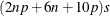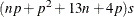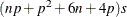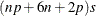The QUANTREG Procedure

Computational Resources

The various algorithms need different amounts of memory for working space. Let p be the number of parameters that are estimated, n be the number of observations that are used in the model estimation, and s be the size (in bytes) of the double data type.

For the simplex algorithm, the minimum working space (in bytes) that is needed isFor the interior point algorithm, the minimum working space (in bytes) that is needed isFor the smoothing algorithm, the minimum working space (in bytes) that is needed isFor the last two algorithms, if you want to use preprocessing, the following additional amount of working space (in bytes) is needed:If sufficient space is available, the input data set is kept in memory. Otherwise, the input data set is reread as necessary, and the execution time of the procedure increases substantially.